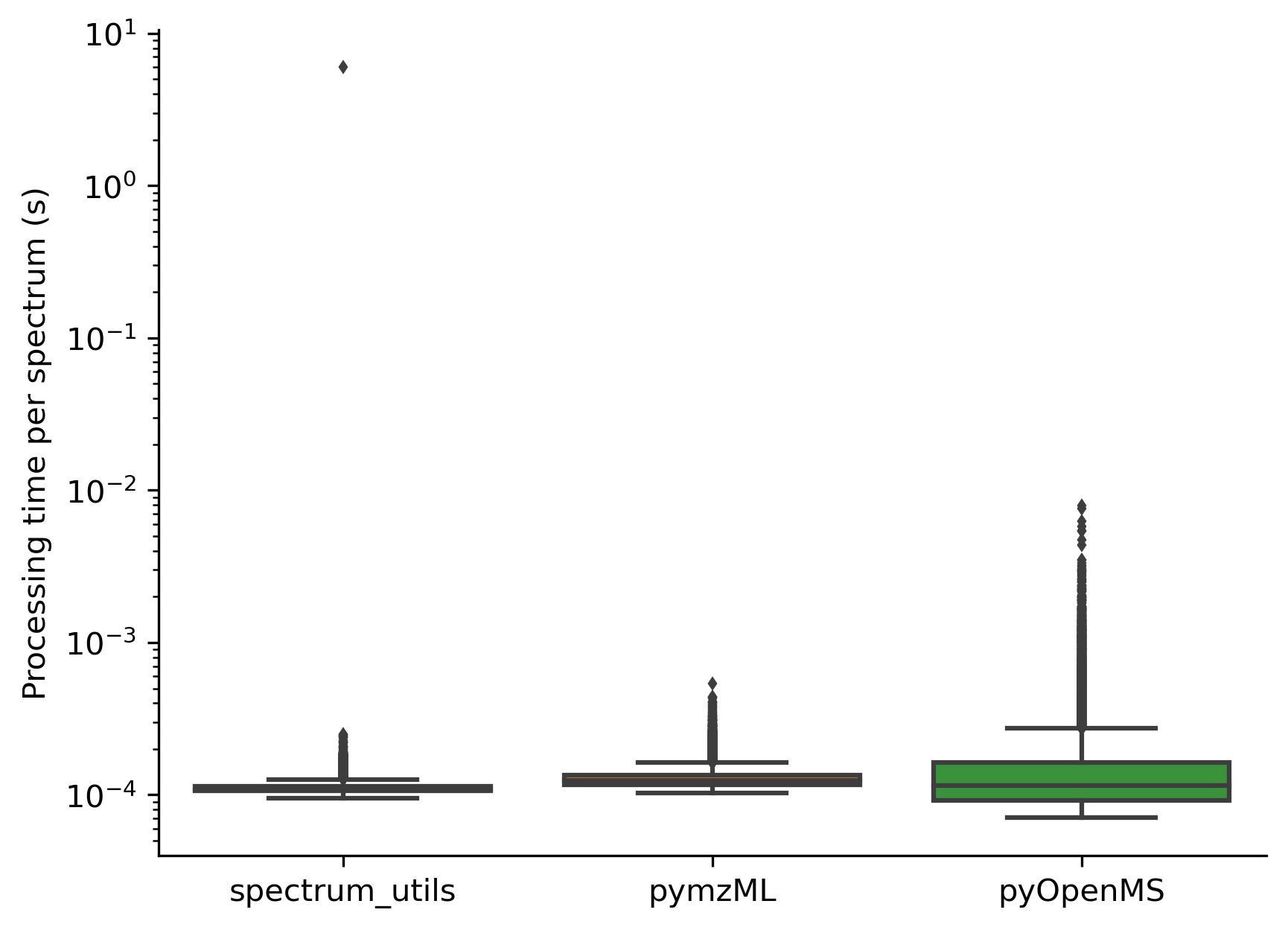# Computational efficiency

Spectrum processing in spectrum_utils has been optimized for computational efficiency using NumPy and Numba to be able to process thousands of spectra per second.

As shown below, spectrum_utils is faster than alternative libraries, such as pymzML (version 2.4.4) and pyOpenMS (version 2.4.0), when performing typical spectrum processing tasks, including the following steps:

• The m/z range is set to 100–1400 m/z.

• The precursor peak is removed.

• Low-intensity noise peaks are removed.

• Peak intensities are scaled by their square root.

```import time

import matplotlib.pyplot as plt
import numpy as np
import pyopenms
import seaborn as sns
import spectrum_utils.spectrum as sus
from pymzml.spec import Spectrum
from pyteomics import mgf

min_peaks = 10
min_mz, max_mz = 100, 1400
fragment_tol_mass, fragment_tol_mode = 0.02, 'Da'
min_intensity = 0.05
max_num_peaks = 150

def time_spectrum_utils(mgf_filename):
runtimes = []
# Omit invalid spectra.
if (len(mgf_in['m/z array']) < min_peaks or
'charge' not in mgf_in['params']):
continue
mz = mgf_in['m/z array']
intensity = mgf_in['intensity array']
retention_time = float(mgf_in['params']['rtinseconds'])
precursor_mz = mgf_in['params']['pepmass']
precursor_charge = mgf_in['params']['charge']
identifier = mgf_in['params']['title']

spectrum = sus.MsmsSpectrum(
identifier, precursor_mz, precursor_charge, mz, intensity,
retention_time=retention_time)

start_time = time.time()

(spectrum.set_mz_range(min_mz, max_mz)
.remove_precursor_peak(fragment_tol_mass, fragment_tol_mode)
.filter_intensity(min_intensity, max_num_peaks)
.scale_intensity(scaling='root', max_intensity=1))

runtimes.append(time.time() - start_time)

return runtimes

def time_pymzml(mgf_filename):
runtimes = []
# Omit invalid spectra.
if (len(mgf_in['m/z array']) < min_peaks or
'charge' not in mgf_in['params']):
continue

spec = Spectrum()
spec.set_peaks(
[*zip(mgf_in['m/z array'], mgf_in['intensity array'])], 'raw')

start_time = time.time()

spec.reduce('raw', (min_mz, max_mz))
spec.remove_precursor_peak()
spec.remove_noise(noise_level=min_intensity)
spec /= np.amax(spec.i)
spec.i = np.sqrt(spec.i)

runtimes.append(time.time() - start_time)

return runtimes

def time_pyopenms(mgf_filename):
experiment = pyopenms.MSExperiment()

runtimes = []
for spectrum in experiment:
# Omit invalid spectra.
if (len(spectrum.get_peaks()) < min_peaks or
spectrum.getPrecursors().getCharge() == 0):
continue

start_time = time.time()

# Set the m/z range.
filtered_mz, filtered_intensity = [], []
for mz, intensity in zip(*spectrum.get_peaks()):
if min_mz <= mz <= max_mz:
filtered_mz.append(mz)
filtered_intensity.append(intensity)
spectrum.set_peaks((filtered_mz, filtered_intensity))
# Remove the precursor peak.
parent_peak_mower = pyopenms.ParentPeakMower()
parent_peak_mower_params = parent_peak_mower.getDefaults()
parent_peak_mower_params.setValue(
b'window_size', fragment_tol_mass, b'')
parent_peak_mower.setParameters(parent_peak_mower_params)
parent_peak_mower.filterSpectrum(spectrum)
# Filter by base peak intensity percentage.
pyopenms.Normalizer().filterSpectrum(spectrum)
threshold_mower = pyopenms.ThresholdMower()
threshold_mower_params = threshold_mower.getDefaults()
threshold_mower_params.setValue(b'threshold', min_intensity, b'')
threshold_mower.setParameters(threshold_mower_params)
threshold_mower.filterSpectrum(spectrum)
# Restrict to the most intense peaks.
n_largest = pyopenms.NLargest()
n_largest_params = n_largest.getDefaults()
n_largest_params.setValue(b'n', max_num_peaks, b'')
n_largest.setParameters(n_largest_params)
n_largest.filterSpectrum(spectrum)
# Scale the peak intensities by their square root and normalize.
pyopenms.SqrtMower().filterSpectrum(spectrum)
pyopenms.Normalizer().filterSpectrum(spectrum)

runtimes.append(time.time() - start_time)

return runtimes

mgf_filename = 'iPRG2012.mgf'
runtimes_spectrum_utils = time_spectrum_utils(mgf_filename)
runtimes_pyopenms = time_pyopenms(mgf_filename)
runtimes_pymzml = time_pymzml(mgf_filename)

fig, ax = plt.subplots()
sns.boxplot(data=[runtimes_spectrum_utils, runtimes_pymzml,
runtimes_pyopenms], flierprops={'markersize': 2}, ax=ax)
ax.set_yscale('log')
ax.xaxis.set_ticklabels(('spectrum_utils', 'pymzML', 'pyOpenMS'))
ax.set_ylabel('Processing time per spectrum (s)')
sns.despine()
plt.show()
plt.close()
```## JIT compilation

Note that the significant outlier for spectrum_utils is caused by Numba’s JIT compilation of the first method call, allowing subsequent calls to be made very efficiently.

If the user knows in advance that only a single method call needs to be made, Numba’s JIT compilation can be disabled to avoid this overhead by setting the `NUMBA_DISABLE_JIT` environment variable to `1`. See the Numba documentation for more information.